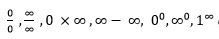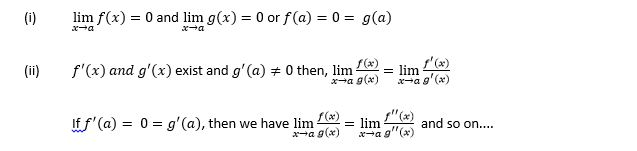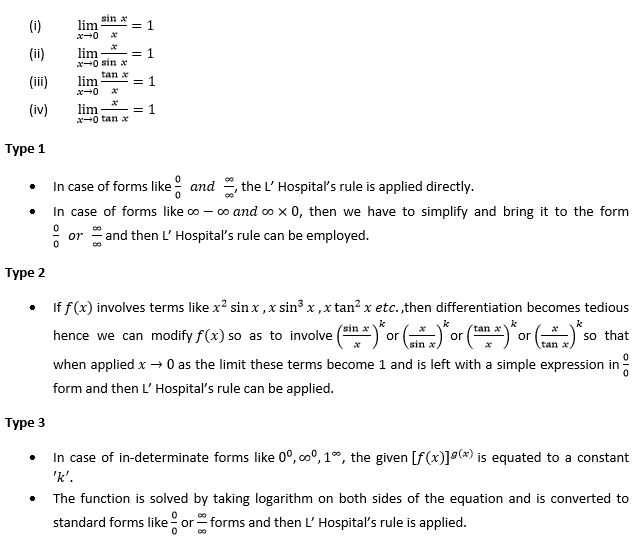## In-determinate Forms

If f(x) at x = a assumes forms likeetc., which do not represent any value are called In-determinate forms. The concept of limit gives a meaningful value for the function  at overcomes these in-determinate forms. The differentiations for such forms are performed using L’Hospital’s (French Mathematician) rule.

## L’ Hospital’s theorem

### Statement

If  f(x) and g(x) are two functions such thatProblems are categorized under three different types.

• Type 1
• Type 2
• Type 3

Note

Standard limits that are used in the problems are